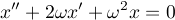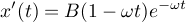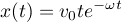## Monday, March 8, 2010

### Landing gear continued

I'll be at the Game Developers Conference this year; if anyone's interested in meeting up drop me a line: mcneja at gmail.

Is there anything so lovely or useful as a critically damped spring?

The basic differential equation:This is saying that the acceleration (the second derivative) is a weighted sum of the velocity (first derivative) and position (x), measured relative to the desired velocity and position. The weights are expressed in terms of a constant omega which indicates the spring's response speed: higher omega means faster movement. The proportions of the weights are chosen to achieve critical damping: the spring returns to its rest position as rapidly as possible without overshooting.

To solve this differential equation we form and solve a characteristic equation:Being critically damped makes the characteristic equation work out very simply. This then allows us to construct the general solution for position as a function of time:The constants A and B are unknowns which we determine via supplying additional constraints on the problem, which will yield a particular solution for our problem. The first constraint I'll impose is that the spring is at rest position at time zero; the idea is that the landing gear is not stretched or compressed at the instant it touches the ground:To solve for the constant B I'll supply a second constraint, which is that the spring is being compressed with speed v0 at time zero. This is the impact velocity. To use this we'll need to differentiate our general solution:Plugging v0 into this yields the answer for B:Now we have an equation for the particular solution of position as a function of time:I have to get off to work now but suffice it to say there's a lot more tedious math where this came from. The next step is to replace omega as an input constant with something more useful: the maximum acceleration we want for the given impact velocity. To find this we have to check the extrema of the acceleration function, as well as the acceleration at time zero. Turns out time zero's acceleration is bigger than any other acceleration by a factor of about 40.

After that I've been working on adding gravity's constant acceleration into the equation which messes things up a fair amount, although the math's not hard.